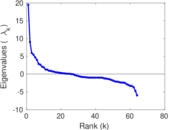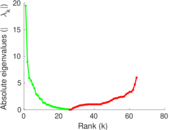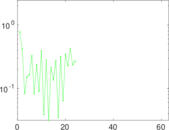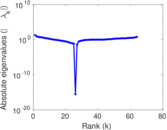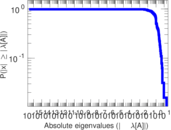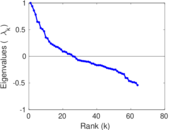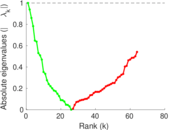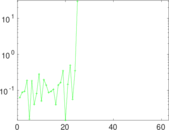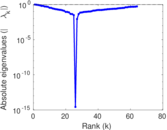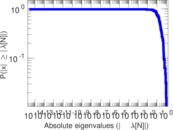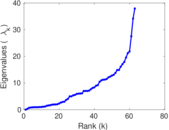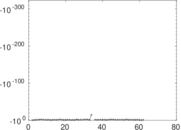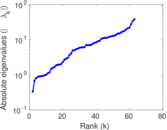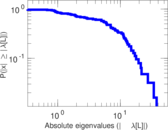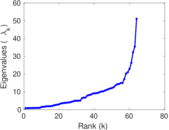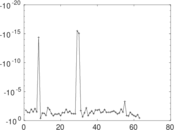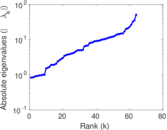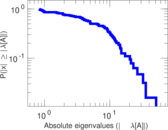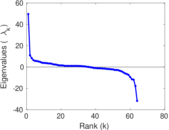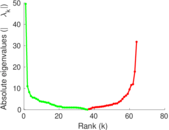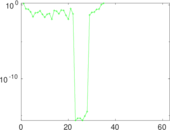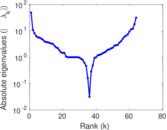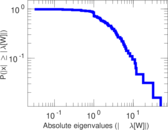# Train bombing

This undirected network contains contacts between suspected terrorists involved in the train bombing of Madrid on March 11, 2004 as reconstructed from newspapers. A node represents a terrorist and an edge between two terrorists shows that there was a contact between the two terroists. The edge weights denote how 'strong' a connection was. This includes friendship and co-participating in training camps or previous attacks.

 Code `Mt` Internal name `moreno_train` Name Train bombing Data source http://moreno.ss.uci.edu/data.html#train AvailabilityDataset is available for download Consistency checkDataset passed all tests Category Human social network Node meaning Terrorist Edge meaning Contact Network format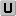Unipartite, undirected Edge type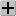Positive weights, no multiple edges LoopsDoes not contain loops

## Statistics

 Size n = 64 Volume m = 243 Loop count l = 0 Wedge count s = 2,818 Claw count z = 14,360 Cross count x = 65,267 Triangle count t = 527 Square count q = 3,672 4-Tour count T4 = 41,134 Maximum degree dmax = 29 Average degree d = 7.593 75 Fill p = 0.120 536 Size of LCC N = 64 Diameter δ = 6 50-Percentile effective diameter δ0.5 = 2.087 61 90-Percentile effective diameter δ0.9 = 3.631 38 Median distance δM = 3 Mean distance δm = 2.627 49 Gini coefficient G = 0.428 305 Balanced inequality ratio P = 0.329 218 Relative edge distribution entropy Her = 0.926 924 Power law exponent γ = 1.602 90 Tail power law exponent γt = 4.041 00 Tail power law exponent with p γ3 = 4.041 00 p-value p = 0.817 000 Degree assortativity ρ = +0.029 464 6 Degree assortativity p-value pρ = 0.516 967 Clustering coefficient c = 0.561 036 Spectral norm α = 19.486 3 Algebraic connectivity a = 0.332 207 Spectral separation |λ1[A] / λ2[A]| = 2.162 47 Non-bipartivity bA = 0.655 074 Normalized non-bipartivity bN = 0.454 523 Algebraic non-bipartivity χ = 0.831 432 Spectral bipartite frustration bK = 0.027 372 3 Controllability C = 2 Relative controllability Cr = 0.031 250 0

## Plots

### Fruchterman–Reingold graph drawing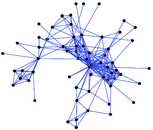### Degree distribution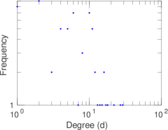### Cumulative degree distribution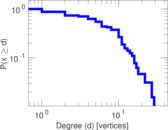### Lorenz curve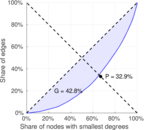### Spectral distribution of the adjacency matrix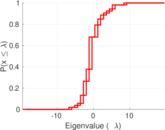### Spectral distribution of the normalized adjacency matrix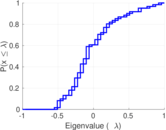### Spectral distribution of the Laplacian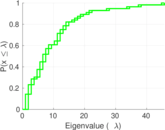### Spectral graph drawing based on the adjacency matrix### Spectral graph drawing based on the Laplacian### Spectral graph drawing based on the normalized adjacency matrix### Degree assortativity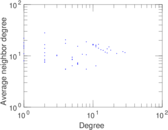### Zipf plot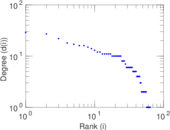### Hop distribution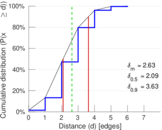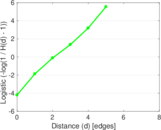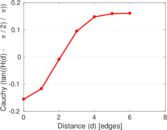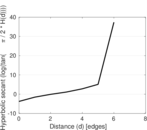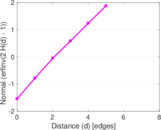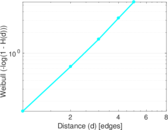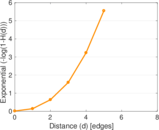### Double Laplacian graph drawing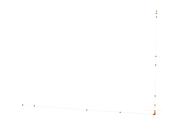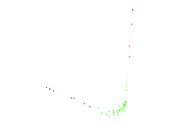### Delaunay graph drawing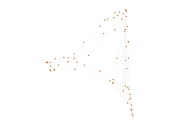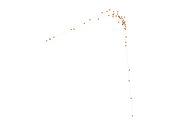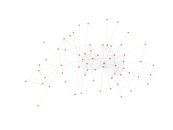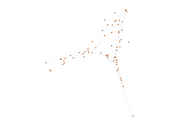### Edge weight/multiplicity distribution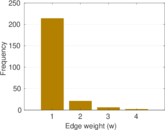### Clustering coefficient distribution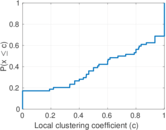### Average neighbor degree distribution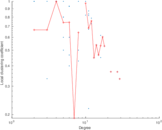### SynGraphy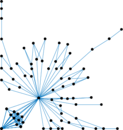### Matrix decompositions plots